Definitions of Square Dance Calls and Concepts
With Confidence [C3B]

Index -->  Plus  |  A1  |  A2  |  C1  |  C2  |  C3A  |  C3B  |  C4  |  NOL  |
Definitions (Text Only) -->  Plus  |  A1  |  A2  |  C1  |  C2  |  C3A  |  C3B  |  C4  |  NOL  |
 Find call:

 \$B8@8l(B\$BJ?9T\$J(B Line \$B\$G(B Ends \$B\$,(B Tandem \$B\$K\$J\$C\$F\$\$\$k\$H\$3\$m(B, \$B\$^\$?\$OB>\$NE,@Z\$J(B formation \$B\$+\$i(B.

Centers \$B\$O(B Hinge \$B\$r\$7\$F(B Very Centers Hinge \$B\$r\$7(B, Ends \$B\$O(B 1/2 Circulate \$B\$r\$7\$^\$9(B. \$B%+%C%W%k\$H\$J\$C\$??M\$O(B step forward \$B\$r\$7(B Two-Faced Line \$B\$N(B Leader \$B\$H\$J\$j(B, \$BB>\$N?M\$O(B Centers U-Turn Back (\$B\$*8_\$\$\$KN%\$l\$k\$h\$&\$K2s\$j(B) \$B\$r\$7(B, Couples Hinge \$B\$N<+J,\$N%Q!<%H\$r9T\$\$\$^\$9(B.

\$BJ?9T\$J(B Two-Faced Line \$B\$G=*\$o\$j\$^\$9(B.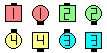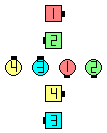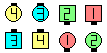With Confidence\$B\$NA0(B Centers Hinge \$B\$H(B Very Centers Hinge as Ends 1/2 Circulate\$B\$N8e(B the Couple steps forwardas The Others do aCenters U-Turn Back& Couples Hinge\$B\$N8e(B (\$B=*\$o\$j(B)

\$BCm
• With Confidence \$B\$OIaDL(B 1 \$B\$DA0\$N%3!<%k\$KIU\$1\$i\$l\$^\$9(B.
• Mini-Wave \$B\$H\$J\$k?M\$O(B, \$B=*\$o\$j\$N(B formation \$B\$NJ}8~\$rG!2?\$K8+Dj\$a\$k\$+\$K\$D\$\$\$F(B, \$B:\$OG\$9\$k\$3\$H\$,\$"\$j\$^\$9(B. \$B\$7\$?\$,\$C\$F(B, \$B%+%C%W%k\$H\$J\$k?M\$O(B, \$BC1\$K(B step forward \$B\$r\$7\$F(B (\$BJ?9T\$J(B Two-Faced Line \$B\$H\$J\$j(B) \$B\$8\$C\$H\$7\$F\$\$\$F(B, \$BB>\$N?M\$N>l=j\$r3NJ]\$9\$k\$3\$H\$,Bg@Z\$G\$9(B.
• \$B1~MQ(B:

• With Confidence But Hold The Wave
• With Confidence But Hold The Two-Faced Line
• Centers start a With Confidence (Galaxy \$B\$+\$i(B)
• Ends start a With Confidence (Twin Diamonds \$B\$+\$i(B)

Short Cycle [C4] (Lee Kopman 1982): Wave \$B\$+\$i(B. 1 \$B\$D\$NF0\$-\$H\$7\$F(B, Centers \$B\$O(B Wave \$B\$N(B Center \$B\$+\$iN%\$l\$k\$h\$&\$K(B U-Turn Back \$B\$r\$7\$F(B, Couples Hinge \$B\$r\$7\$^\$9(B. Two-Faced Line \$B\$G=*\$o\$j\$^\$9(B.

Short Cycle: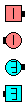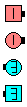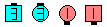Short Cycle\$B\$NA0(B Centers U-Turn Back(by turning away from the Center)\$B\$N8e(B Couples Hinge\$B\$N8e(B (\$B=*\$o\$j(B)Choreography for With ConfidenceComments? Questions? Suggestions?

https://www.ceder.net/def/withconfidence.php?language=japan&level=master
06-April-2020 01:53:14